QUESTION #544

# What is the electric field due to an infinite sheet of charge?

Let us first consider the electric field on the axis of a uniformly charged ring - you will see why this is relevant soon. This situation is shown in the diagram: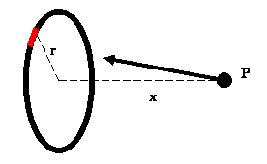We know that the net electric field will point towards the centre of the ring, since all vertical components will cancel out due to the symmetry of the shape. This means we need only consider the components in the direction of the ring. First we will consider the force on particle P due to the red element highlighted. By Coulombs law we know that the contribution to the field will be: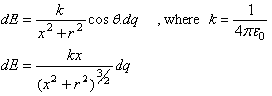Since all the terms are constant this means that the total electric field due to the ring will be: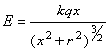Now we will consider the problem of the infinite sheet. We think of the sheet as being composed of an infinite number of rings. We will let the charge per unit area equal sigma: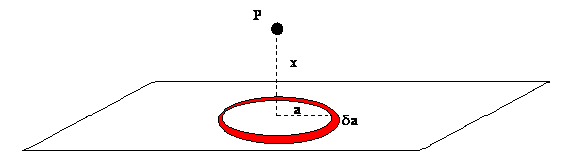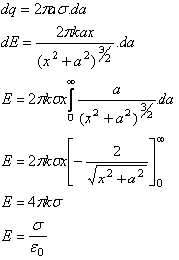This is a very interesting result as the distance from the plane does not affect the electric field produced by it.